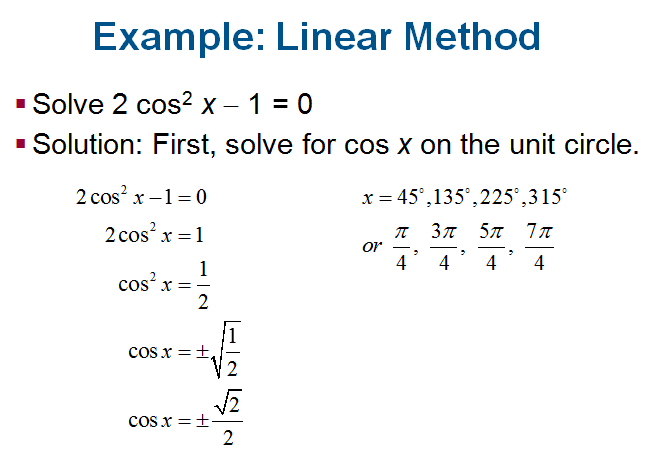# SCC Education

## Solving Trigonometric Equations

1 Solving Trigonometric Equations
2 More on Trigonometric Equations
3 Trigonometric Equations Involving Multiples Angles
4 Parametric Equations and Further Graphing

1 Solving Trigonometric Equations

§Decide whether the equation is linear or quadratic in form, so you can determine the
solution method.
§If only one trigonometric function is present, first solve the equation for that function.
§If more than one trigonometric function is present, rearrange the equation so that one side
equals 0. Then try to factor and set each factor equal to 0 to solve.
§If the equation is quadratic in form, but not factorable, use the quadratic formula.
Check that solutions are in the desired interval.

Try using identities to change the form of the equation. If may be helpful to square both
sides of the equation first. If this is done, check for extraneous solutions